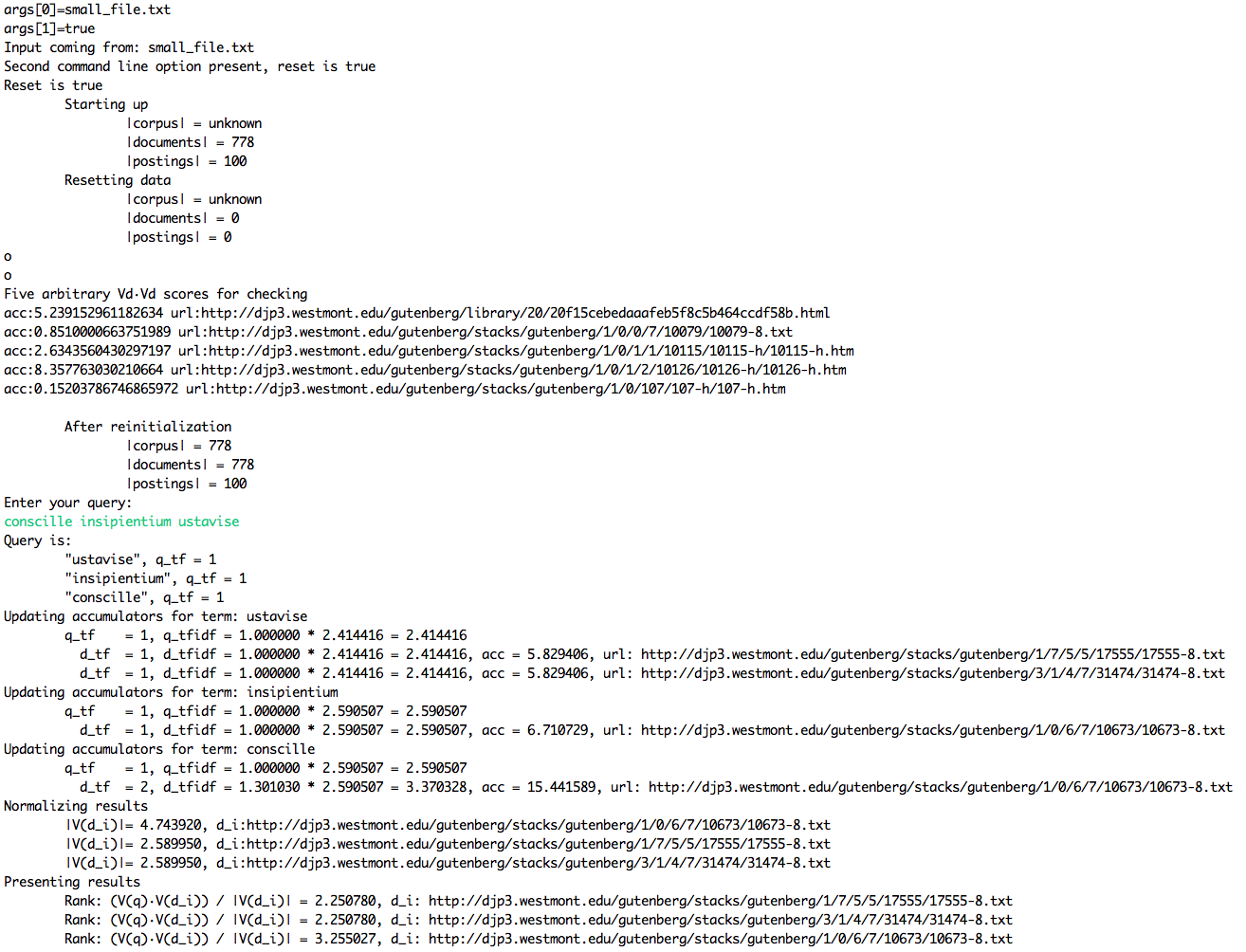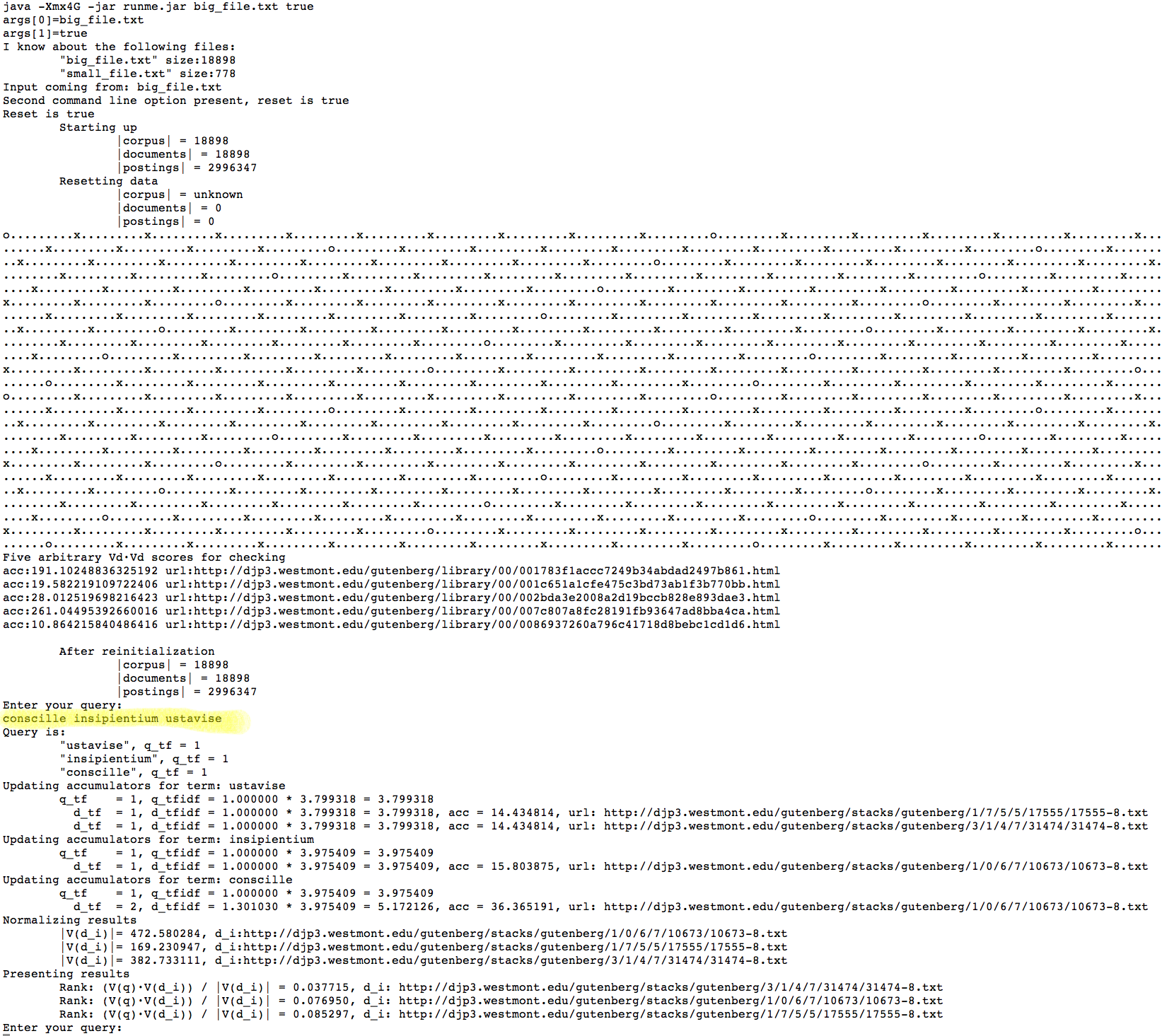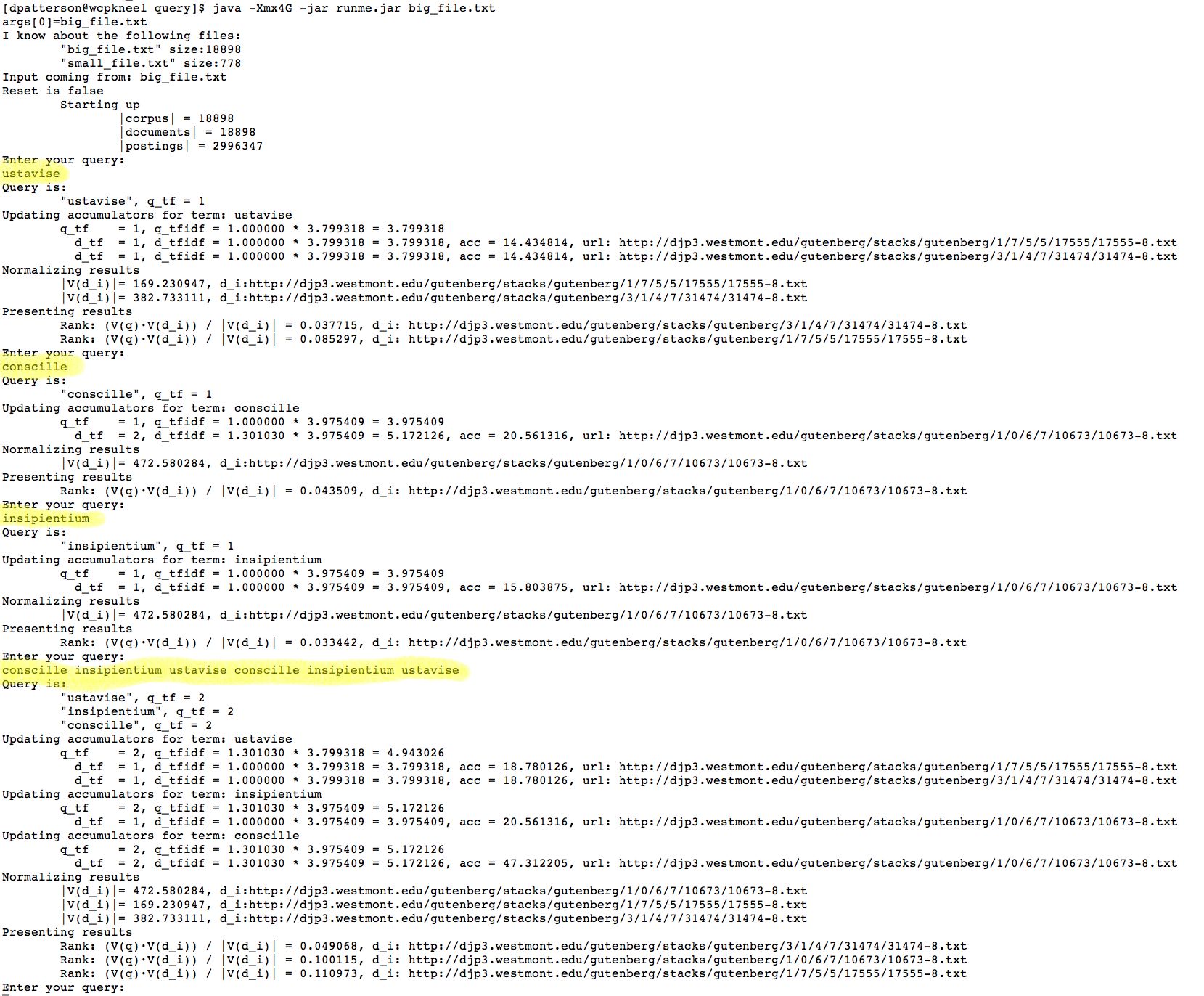## Execute a query using ranked retrieval

• Goals:
1. To teach you how to use the crawl and posting list to answer a user's query.
2. To understand the efficient cosine ranking algorithm
• Groups: This can be done in groups of 1 or 2.
• Discussion: Use Piazza for general questions whose answers can benefit you and everyone.
• Write the necessary programs to answer a query:
• Input: A posting list generated from the project gutenberg crawl.
• Here is a small sample one: small file
• Here is the full posting list: big file
• Format: The first element is a term. The second element is a JSON-parsable list of urls and term frequencies
• Evaluation: Each person (even if you are in a group) will take a Eureka quiz (TBD) which will ask 10 questions of the form:
• What is the {first...fifth}-most relevant document (by id number) for the query X and what is it's relevance score?
group members may assist each other if they want, but are not required to.
• Guides: V1
• Here is how I went about solving this:
1. First set up a project that includes BerkeleyDB and a JSON parser library
2. Write code to calculate the number of documents in the input file. (This only has to be done once per input file if you store the number somewhere)
3. Create a BerkeleyDB table to store (url, double) pairs: your document accumulators
4. Write code to calculate the document vector lengths V_d * V_d (this only has to be done once per input file if you store the results in your BerkeleyDB)
5. Write code to ask for a query
6. Use the efficient cosine similarity accumulator algorithm to calculate V_q * V_d
7. Normalize the similarity scores by the document vector length
8. Sort the the most relevant documents for a given input query. Highest score is the most relevant because it's the cosine of the angle between them (or proportional in my case)
9. Ask for a new query
• Here is a screen shot of my output. The movie is to show speed•• To get these numbers I used log10. Getting the exact numbers correct is important.
• Remember that the query counts as a document and will bump up your corpus size and document frequency by one when finding the elements in V_q
• Evaluation:
1. Correctness: Did you get the right answer?
2. Due date: 12/04 11:59pm No late credit
3. This is an assigment grade